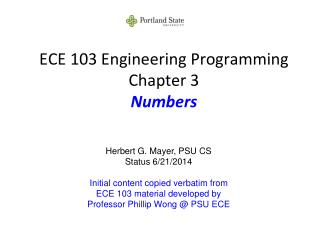Download PresentationECE 103 Engineering Programming Chapter 3 Numbers

# ECE 103 Engineering Programming Chapter 3 Numbers - PowerPoint PPT PresentationDownload Presentation## ECE 103 Engineering Programming Chapter 3 Numbers

- - - - - - - - - - - - - - - - - - - - - - - - - - - E N D - - - - - - - - - - - - - - - - - - - - - - - - - - -
##### Presentation Transcript

1. ECE 103 Engineering ProgrammingChapter 3Numbers Herbert G. Mayer, PSU CS Status 6/21/2014 Initial content copied verbatim from ECE 103 material developed by Professor Phillip Wong @ PSU ECE

2. Syllabus • What’s This Blue Code? • Binary Numbers • Number Conversion Decimal - Binary • Bitwise Operations • Logic Operations • Other Base Representations • Convert Decimal to Hex • Positive and Negative Integers • Floating Point Numbers

3. What’s This Blue Code? void foo( void ) { // foo } //end foo // <- implied return here int main( /* no params */ ) { // main foo(); return 0; // says: nothing went wrong! } //end main // learned about: Functions // similar to the printf() which you saw earlier

4. Binary Numbers Bit  Smallest unit of information(binary digit) A single bit has two distinct states: 0 (logical False, power close to 0 V 1 logical True, power close to defined + V A binary number consists of n bitsgrouped together. LSB MSB bn-1bn-2…b1b0 = bn-12n-1 + bn-22n-2 + … + b121 + b020 3

5. Binary Numbers Table 1: Given n bits, the number of possible states = 2n 4

6. Number Conversion Decimal - Binary Convert from binary to its equivalent base 10 value Expand the powers of two Example: What is 11102in decimal? 11102 = (123) + (122) + (121) + (020) = (18) + (14) + (12) + (01) = 1410 Convert from base 10 to its equivalent binary value Successively divide by two; keep track of remainders Example: What is 1410 in binary? Read the remainders backwards. Hence, 1410 = 11102 5

7. Bitwise Operations Bitwise Complement Bitwise AND Bitwise OR Bitwise XOR Bitwise Addition 6

8. Logic Operations Logic operations are done one bit at a time (unary, AKA monadic) or a pair of bits (binary, AKA dyadic) Example: ~1011 = 0100 Complement, unary 1010 & 1100 = 1000 Bitwise AND, binary 1010 | 1100 = 1110 Bitwise OR 1010 ^ 1100 = 0110 Bitwise XOR 7

9. Other Base Representations Octal (base 8  0, …, 7) Hexadecimal (base 16  0, …, 9, A, B, C, D, E, F with F representing 1510) Table 2: 4-bit positive integer conversion table 8

10. Convert Binary to Hex Converting from binary to its equivalent hex:1) Separate binary value into 4-bit groups2) Replace each group by its hex value Example: 4410010 = 10101100010001002 = AC4416 Converting from hex to its equivalent binary:Replace each hex value by its 4-bit binary value. Example: 2741110 = 6B1316 = 01101011000100112 9

11. Positive and Negative Integers Integers are exactly representable in base 2 Table 3: 4-bit positive only values 0 to 15 If only positive integers and zero are needed, then all of the bits in the binary representation are available to express the value. Given : n bits Range: 0 to 2n – 1 10

12. For negative integers, the most significant bit (MSB) of the binary value is reserved as a sign bit Negative values are expressed as 2’s complement Table 4: 4-bit positive and negative values -8 to +7 If both positive and negative integers are needed, the maximum positive value is reduced by a factor of 2 Given : n bits Range: –2n-1 to 2n-1 – 1 11

13. Floating Point Numbers God created integers; Man invented floats Floating point is used to express real-valued numbers. There is an implicit base and decimal point Example: 2.0 3.1415 –634.9 Example: In scientific notation format (base 10) –6.349 × 102 mantissa exponent sign base 12

14. Binary can be used to represent floating point values, but usually only as an approximation IEEE 754single-precision (32-bit) standard s e1e2…e8 b1b2…b23 8 bits Interpreted as unsigned integer e' 1 bit Sign 0→+ 1→– 23 bits Interpreted as a base 2 value defined as m' =0.b1b2…b23 = b12-1 + b22-2 +…+ b232-23 if e'≠ 0 then FP number = (-1)s × (1 + m') × 2e'-127 if e' = 0 then FP number = (-1)s × m'× 2-126 13

15. Example: IEEE 754 single precision (32-bit) 01010110010010100000000000000000 The more bits available, the more precise the mantissa and the larger the exponent range Number = (-1)s × (1 + m') × 2e'-127 = 1.578125 × 245≈ 5.55253372027 × 1013 s = 0 m' = 2-1 + 2-4 + 2-6 = 0.578125 e' = 17210 14